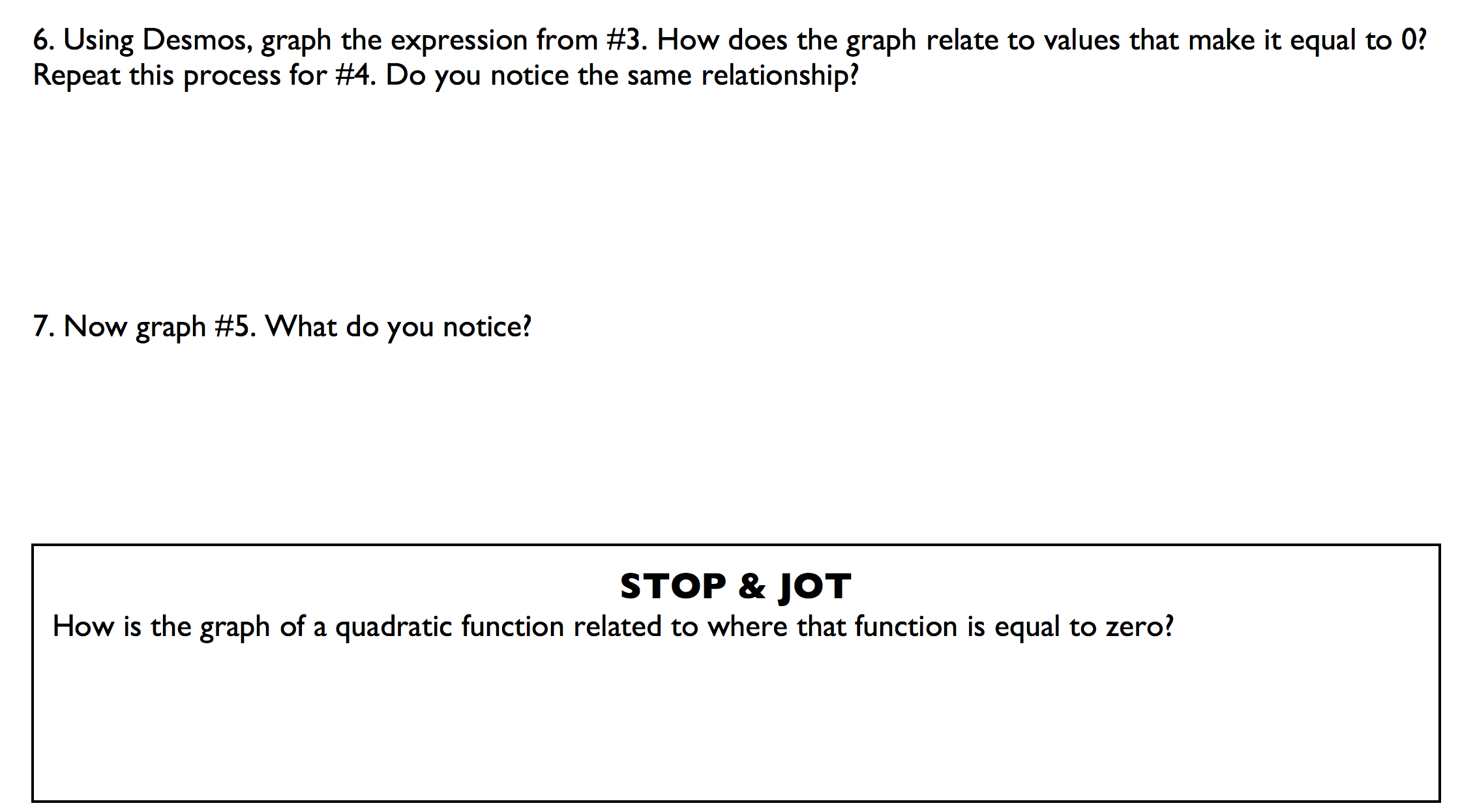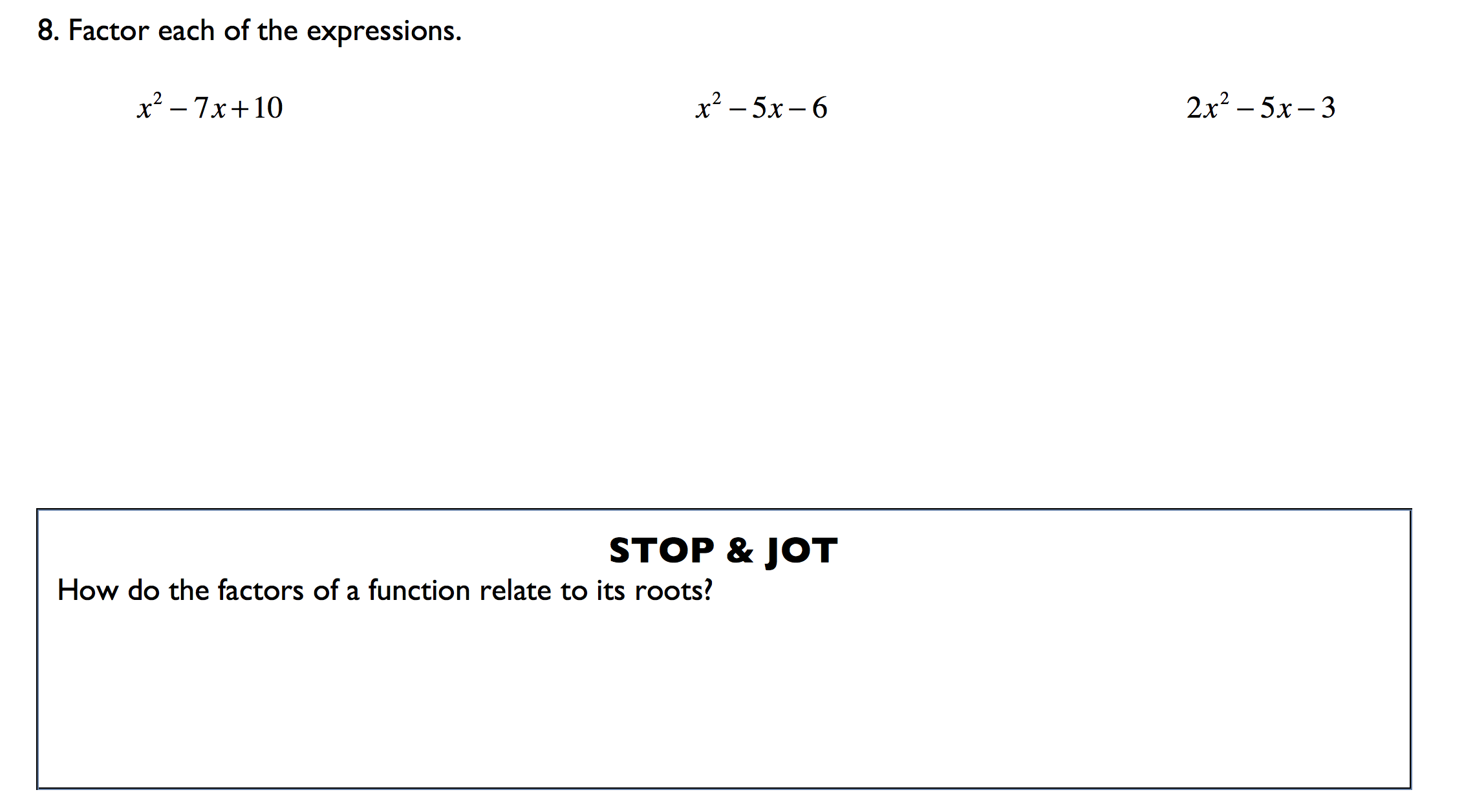## Exploring the roots of quadratic functions

In my algebra 2/trigonometry class, I wanted to spend a day with them exploring quadratic functions, their roots, factors, and how everything is related. This is taught in algebra 1, but the students always seem forget all this after geometry. It’s a perfect intro to our quadratics unit.

Part 1. Building off Dan Meyer’s approach to factoring trinomials, I first had the students find all values that make quadratic expressions equal to 0.Most found this straightforward and doable, which was great because that was the point: accessibility. The third expression had one non-integer value as an answer, which I included on purpose to throw them off. A couple kids actually factored and used the zero-product property, which was ahead of the game…they actually remembered something from algebra 1!

Part 2. I had them use Desmos to examine the graphs of the three functions and find a relationship between the graphs and the values they found that made each equal 0. It took several minutes, but there were definitely some ah-ha! moments when they saw the connection, which was cool.I then re-introduced them to be the term “roots” as a way of describing these magic numbers.

Part 3. I also wanted them explore the relationship between the factors and the roots. Because of time, we more or less did this together (instead of them working it out in groups). We first factored all the expressions. I then asked how the factors relate to the roots of each function. Most of the class realized that when each factor is set to 0, the roots result from these “mini” equations.Overall, the lesson was solid. I really liked that, other than imparting the term “root,” there was no need for me to lead any part of the lesson. I simply provided resources and asked the right questions that spurred deep thinking.

Exit slips showed their understanding of the connection between the roots and factors wasn’t strong. This was probably due to the fact that the lesson was a bit rushed at that point. The next lesson focuses strictly on finding roots by means of factoring quadratic equations, so hopefully that helps. I also felt the lesson flip-flopped around the term expression and function too much. Leaving the lesson, the difference between the two could have been unclear and may cause some confusion amongst the kiddos. Another thing I would have changed is not having all trinomials…the kids could possibly generalize that all quadratic functions are trinomials, which is obviously not true. Even if they don’t go that far, a variety quadratic functions still would have been better for them to explore.

Here is the document.

bp